Conge 精进

# Reinforcement Learning 第六周课程笔记

2015-09-26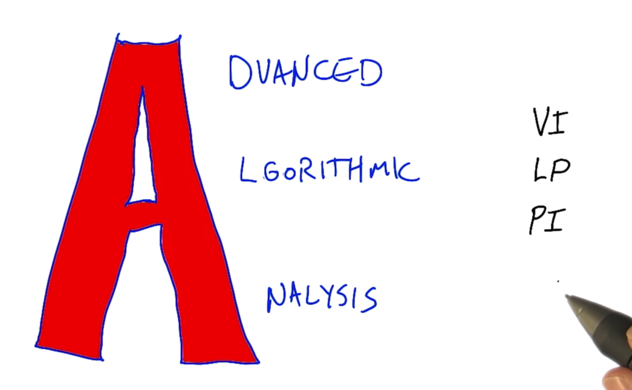## Value iteration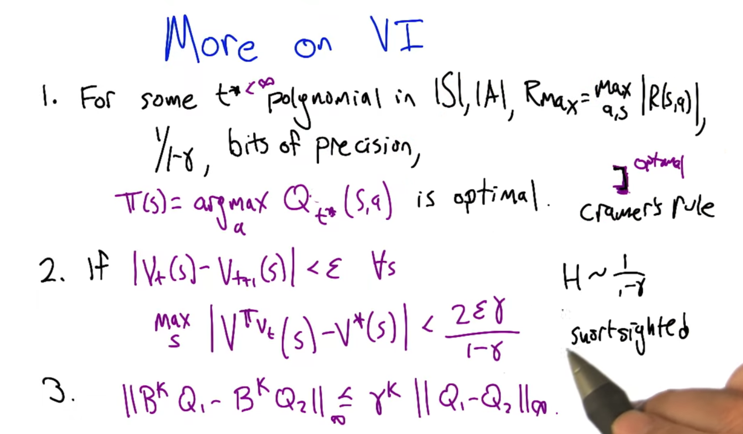• 1 tells us that VI converges in a reasonable amount of time t* (finite). We know t* exists, but we don’t know when that will happen.

• 2 Gives us an bound of difference between the policy now and optimal policy. We can take this bound and test when it is a decent time to stop VI and take the current policy.

• Both 1 and 2 encourage us choosing a small γ. (γ tells us how further in the future we should look). The effective horizon is H ~ 1/(1-γ).

• 3 applying Bellman Operator K times to Q functions will shrink the distance between Q functions.

## Linear Programming

• Only one way to solve MDP in polynomile time：solving Bellman equation through Linear Programming. A linear programming problem may be defined as the problem of maximizing or minimizing a linear function subject to linear constraints. ( Defination extracted form this PDF)

• Bellman equation has one part that is not linear, the max function. But it can be expressed by a series of linear function and a objective function `min`.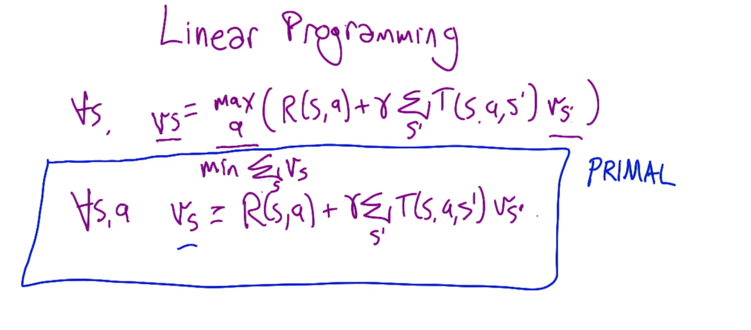• in the primal, the objective function is the minimum of sum of all the Vs;
• in linear programming, we can change constraints to variables and variables to constraints, and the resulting linear program is equivalent to the old one.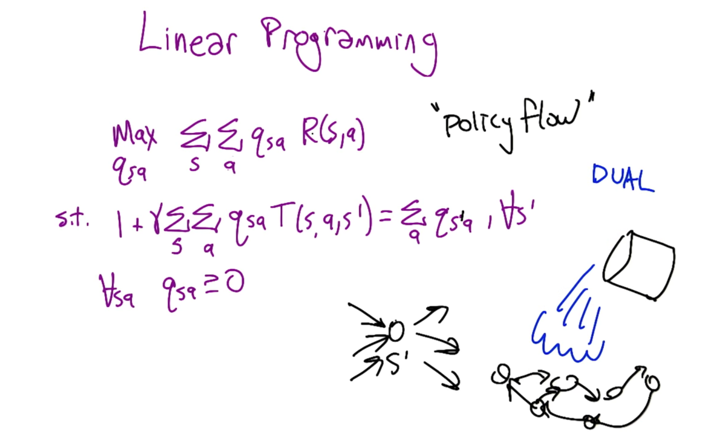• Dual is a new linear program comes from the old primal version of linear program (没有推导过程）
• qsa is “Policy flow”, maximize the expected rewards of all states.
• For each possible next state, we wanted it to be true that the amount of policy flows going through the next state should be equal to the number of the state it has been visited.

## Policy Iteration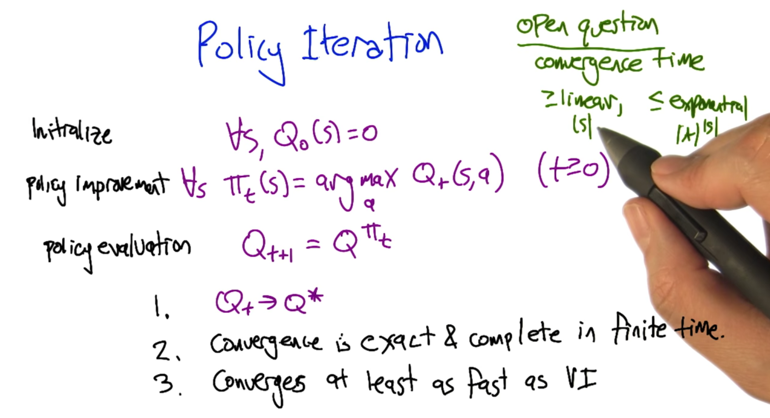• Initialize the first step Q value of all the states to be 0; improve the policy at time t; Apply the policy to calculate the Q value of t+1 step.
• Convergence time is an open question (but it is finite): >= linear, <= exponential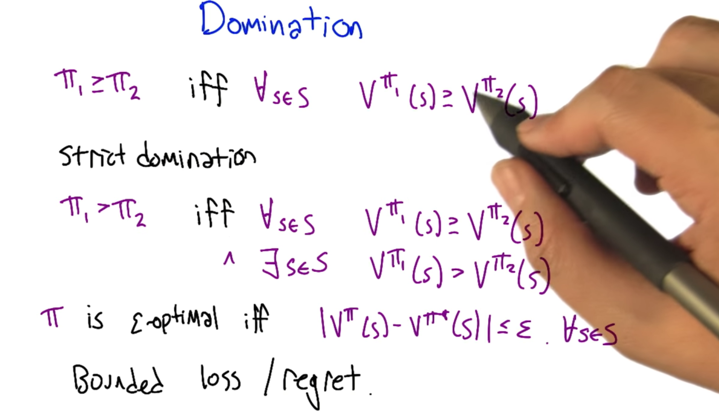• For every state, if the value of it follows π1 always equals or is larger than the value when it follows π2, we say π1 dominates π2.
• if π1 dominates π2 and there exits states that Vπ1(s) > Vπ2(s), we say π1 strict dominates π2.
• if for every state, the distance between the value following policy π and following the optimal policy π* is no larger than ε, then π is ε-optimal.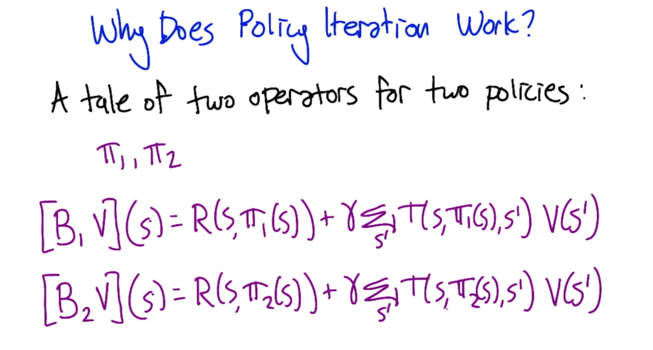• B1 makes the update follows π1 and B2 makes the update follows π2
• Applying B operator to two Values will not make them further apart, if the two values are the same, applying B will not make them closer.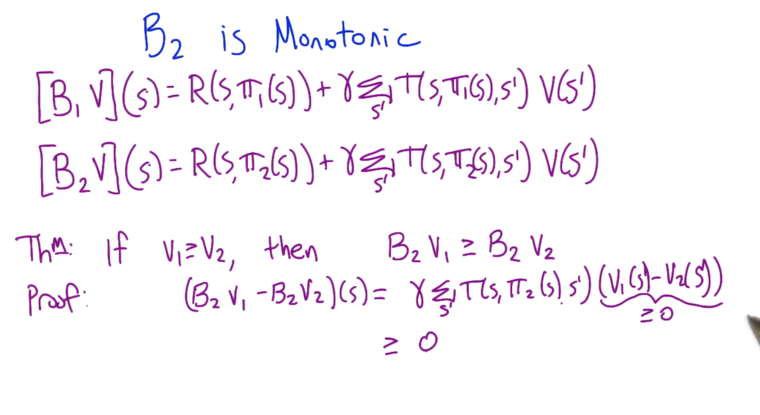• Theorem: if V1 dominates V2, applying B will keep the ordering: BV1 >= BV2.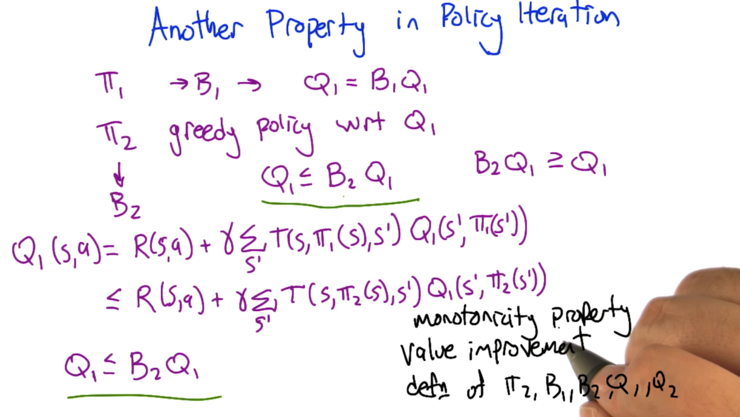• Q1 is the fix point of B1;
• π2 is greedy policy with respect to Q1
• B2 is the Bellman operator of π2
• Applying B2 (the greedy function wrt Q1) on </sub>Q1 will add a bound to Q1, thus getting a better Q1. This is called Value Improvement.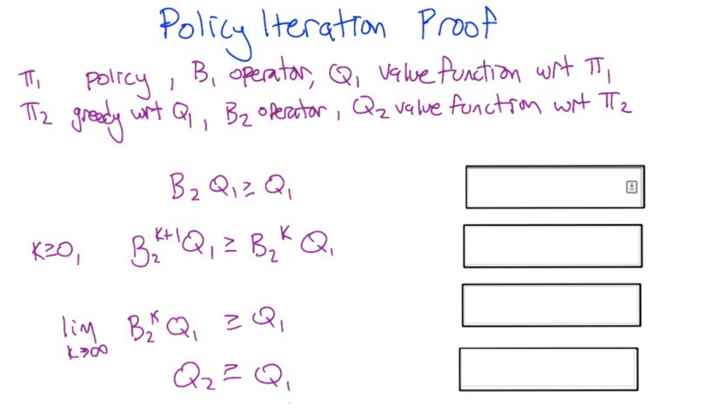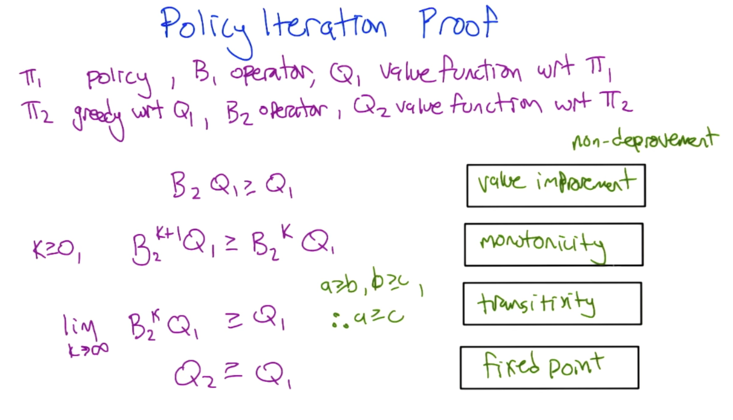• Value improvement (or value non deprovement): for each state, value will improved until it could not get better anymore.
• Monotonicity: Value can only get better after each iteration.
• Transitivity: a >= b, b >=c, so a >=c.
• Fixed point. If we apply B2 over and over again on Q1, we will reach the fixed point of B2,which is Q2.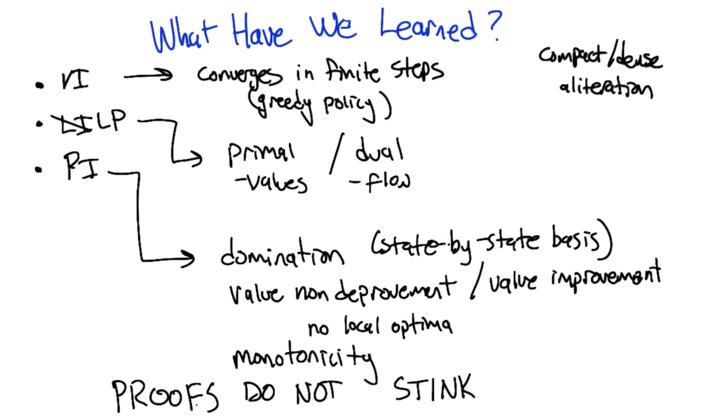• In Value iteration, greedy policy converges in finite step. This does not necessarily mean value function will converge.
``````2015-09-23 初稿
2015-09-26 完成
2015-12-04 reviewed and revised.
``````